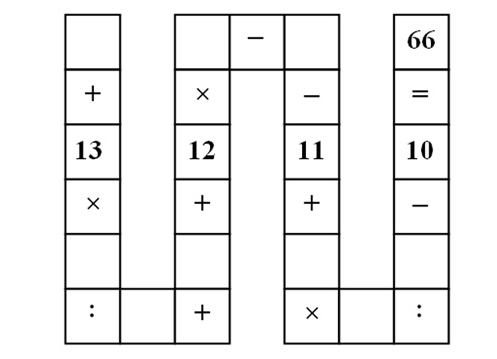## brute force arithmetic

Came across this puzzle today. Instead of trying to do some fancy multivariate analysis, here's a brute force solution.``````"""
Solve the `nam puzzle' using brute force.

See http://goo.gl/8b0ri6 for full problem description.
"""

def main():
from itertools import permutations
for p in permutations(range(1, 10)):
a, b, c, d, e, f, g, h, i = p
if a + ((13 * b) / c) + d + (12 * e) \
- f - 11 + ((g * h) / i) - 10 == 66:
print ':) => {}'.format(p)
break
else:
print ':('

if __name__ == '__main__':
import sys
sys.exit(main())
``````
``````l00k:~/ws/20150522\$ python nam_puzzle.py
:) => (1, 2, 3, 4, 6, 5, 7, 9, 8)
``````